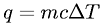Equations > Chemistry > Thermochemistry > Specific Heat Capacity to Heat Equation

Specific Heat Capacity to Heat EquationLatex Code:

MathML Code:

 $q=\mathrm{mc}\Delta T$

MathType 5.0: